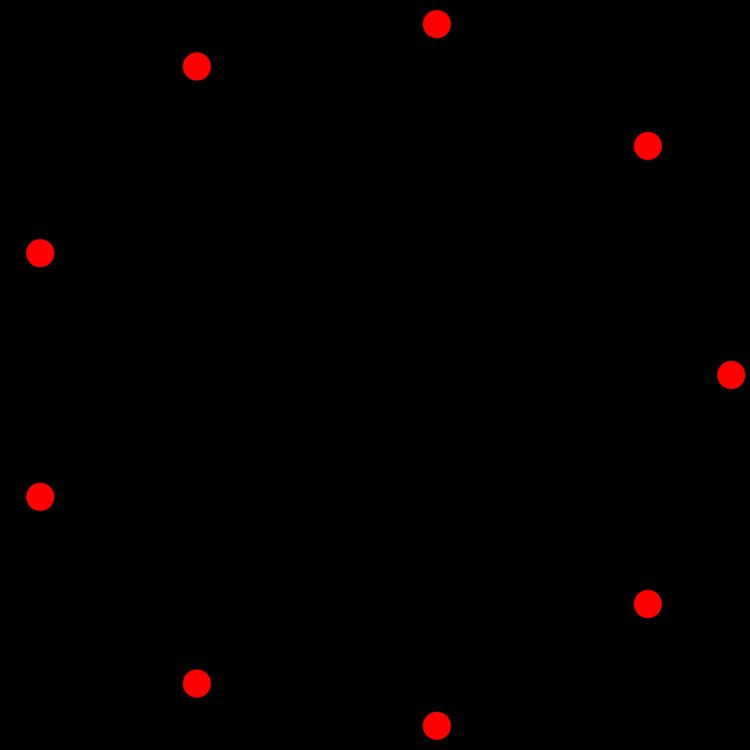A8 polytope

Updated onIn 8-dimensional geometry, there are 135 uniform polytopes with A8 symmetry. There is one self-dual regular form, the 8-simplex with 9 vertices.

Each can be visualized as symmetric orthographic projections in Coxeter planes of the A8 Coxeter group, and other subgroups.

Graphs

Symmetric orthographic projections of these 135 polytopes can be made in the A8, A7, A6, A5, A4, A3, A2 Coxeter planes. Ak has [k+1] symmetry.

These 135 polytopes are each shown in these 7 symmetry planes, with vertices and edges drawn, and vertices colored by the number of overlapping vertices in each projective position.

References

Similar Topics
Pete and Repeat
Phelim Boyle
Jelle ten Rouwelaar
Topics